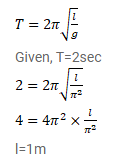# A pendulum having time period equal to two seconds is called a seconds pendulum.

Question:

A pendulum having time period equal to two seconds is called a seconds pendulum. Those used in pendulum clocks are of this type. Find the length of a seconds pendulum at a place where $g=\pi^{2} \mathrm{~m} / \mathrm{s}^{2}$.

Solution: